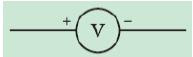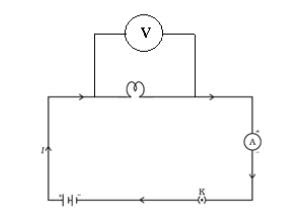## ELECTRIC POTENTIAL

The movement of electrons in a metal wire takes place only if there is some sort of difference in electric pressure – called the potential difference – along the conductor. This difference of potential may be produced by a battery. The chemical action within a cell generates the potential difference across the terminals of the cell, even when no current is drawn from it. When the cell is connected to a conductor, the potential difference sets the charges in motion in the conductor and produces an electric current.

## Definition of Electric Potential:

We define the electric potential difference between two points in an electric circuit as the work done to move a unit charge from one point to the other.

## Formula:

$\textrm{Potential difference between two points} = \frac{work\ done}{charge}$
$V=\frac{V}{Q}$

## SI unit:

The SI unit of electric potential difference is volt (V), named after Alessandro Volta.

## Definition of one volt:

One volt is the potential difference between two points when 1 joule of work is done to move a charge of 1 coulomb from one point to the other.
Therefore,

$1 volt=\frac{1 joule}{1 coulomb}$

## Device used to measure potential difference:

The potential difference is measured by means of an instrument called the voltmeter.

## Symbol in an electric circuit:## Connection:

The voltmeter is always connected in parallel across the points between which the potential difference is to be measured.1. Name a device that helps to maintain potential difference across a conductor.

2. What is meant by saying that potential difference between two points is 1V?

3. Write the relation which states the relation between potential difference and work done.

4. How much energy is given to each coulomb of charge passing through a 6V battery?

5. How much work is done in moving a charge of 2 C across two points having a potential difference of 12 V?

6. Name the device that measures the potential difference across two points in an electric circuit. How it is connected in an electric circuit?